CS 440/ECE 448
Margaret Fleck

## Recurrent Neural Nets

Recurrent neural nets (RNNs) are neural nets that have connections that loop back from a layer to the same layer. The RNN shown below has a single hidden layer. We can think of the self-connected layer as containing multiple processing units, similar to the hidden layer of a normal neural network. The intent of the feedback loop in the picture is that each unit is connected to all the other units in the layer.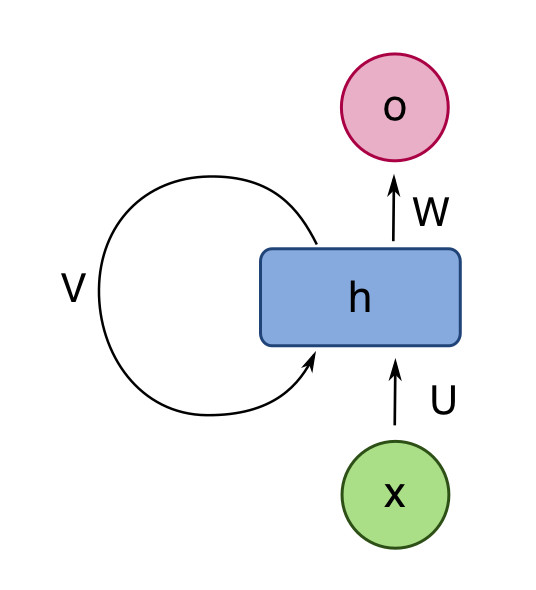from Wikipedia

Alternatively, we can think of an RNN as a state machine. That is, we think of the values from all the units in the layer as bundled up into a state vector, $$s_i$$ in the picture below. At each timestep, the RNN reads an input vector and the current state. It produces an output vector and a new state, using a state transition function (R), an output function (O), and some tunable parameters $$\theta$$.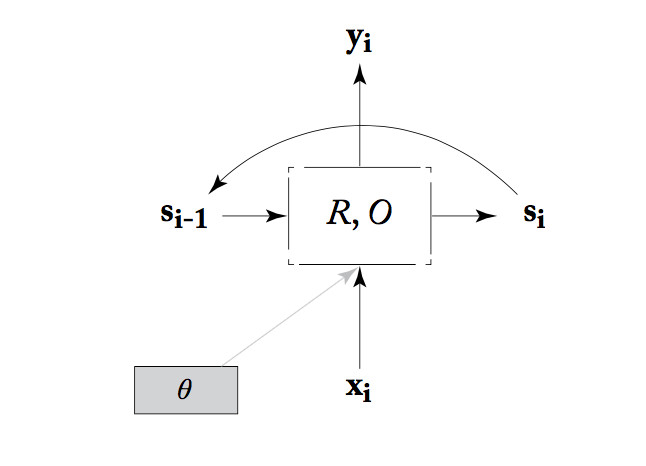from Yoav Goldberg

To see how computation proceeds and, more importantly, how training works, we unroll the RNN. That is, we make a clone of the RNN for each timestep, creating the diagram below. Notice that all copies of the unit share the same parameter values.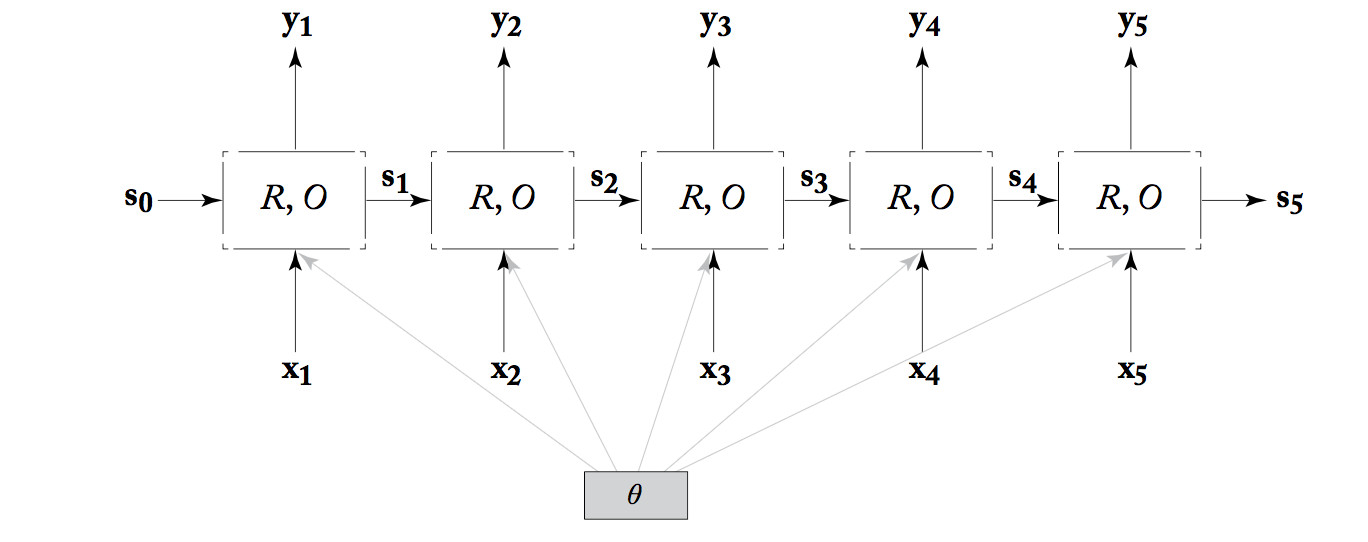from Yoav Goldberg

An RNN can be used as a classifier. That is, the system using the RNN only cares about the output from the last timestep, as shown below. In an NLP system, the final output value may actually be complex, e.g. a summary of an entire sentence. Either way, the final output value is fed into a later processing that provides feedback about its performance (the loss value).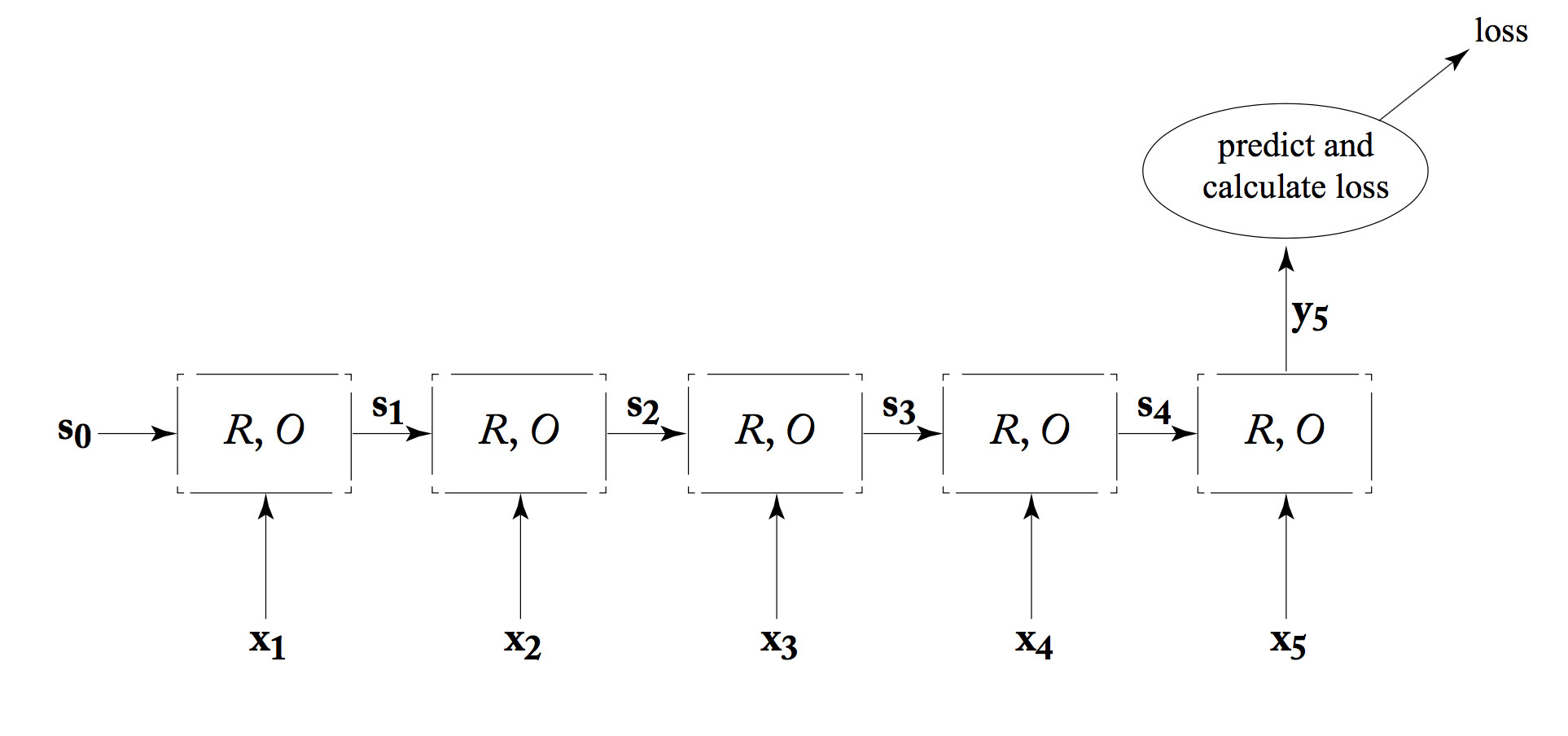from Yoav Goldberg

This kind of RNN can be trained in much the same way as a standard ("feedforward") neural net. However, values and error signals propagate in the time direction. The forward pass calculates values moving to the right. Backpropagation starts at the final loss node and moves back to the left. This is often called "backpropagation through time."

Like convolutional layers, RNNs rarely do an entire task by themselves. They are typically combined with other processing layers, either other types of neural net machinery or non-neural processing.

## Other types of RNNs

RNNs have proved most useful for modelling sequential data, which is common in natural language understanding and generation tasks. Some of these tasks use variations on the classifier design shown above. For example, many tasks require mapping the input sequence into a corresponding output sequence, e.g. mapping words to part-of-speech tags. In this case, we would care about getting the correct output at each timestep (not just the final one). So the connection to our loss function would look like this: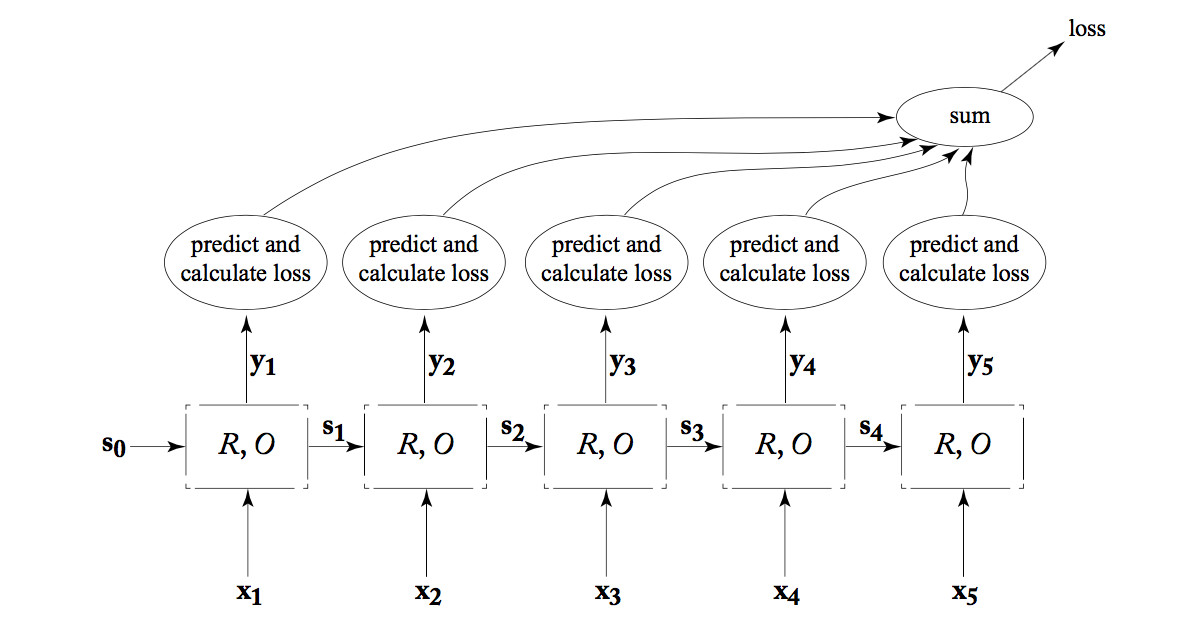from Yoav Goldberg

We can join two RNN's together into a "bidirectional RNN." One RNN works forwards from the start of the input, the second works backwards from the end of the input. The output at each position is the concatenation of the two RNN outputs. This combined output would typically receive further processing (not show) and then eventually be evaluated to produce a loss. When the loss information propagaes backwards, both RNNs receive feedback based on their joint answer.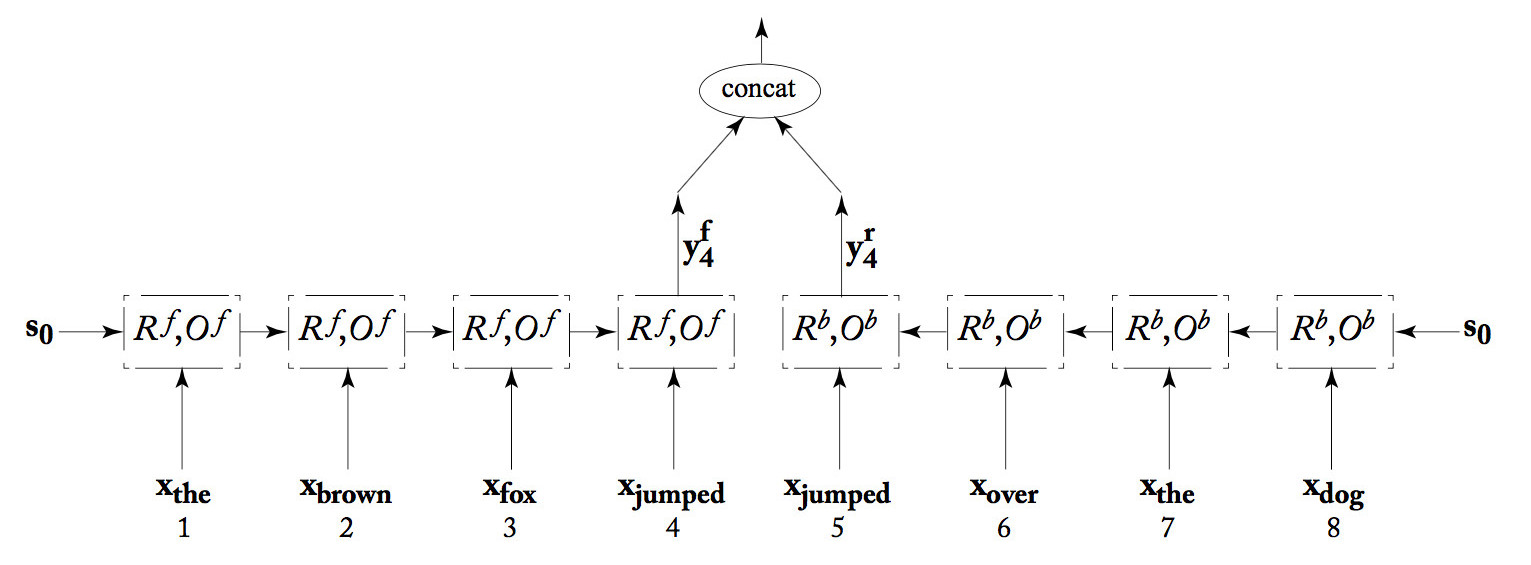from Yoav Goldberg

We can also build "deep" RNN's, which have more than one processing layer between the input and output streams. The one shown below has three layers. Because each processing unit in an RNN is already complex (equivalent to multiple units in a standard neural net), it's unusual to see many layers.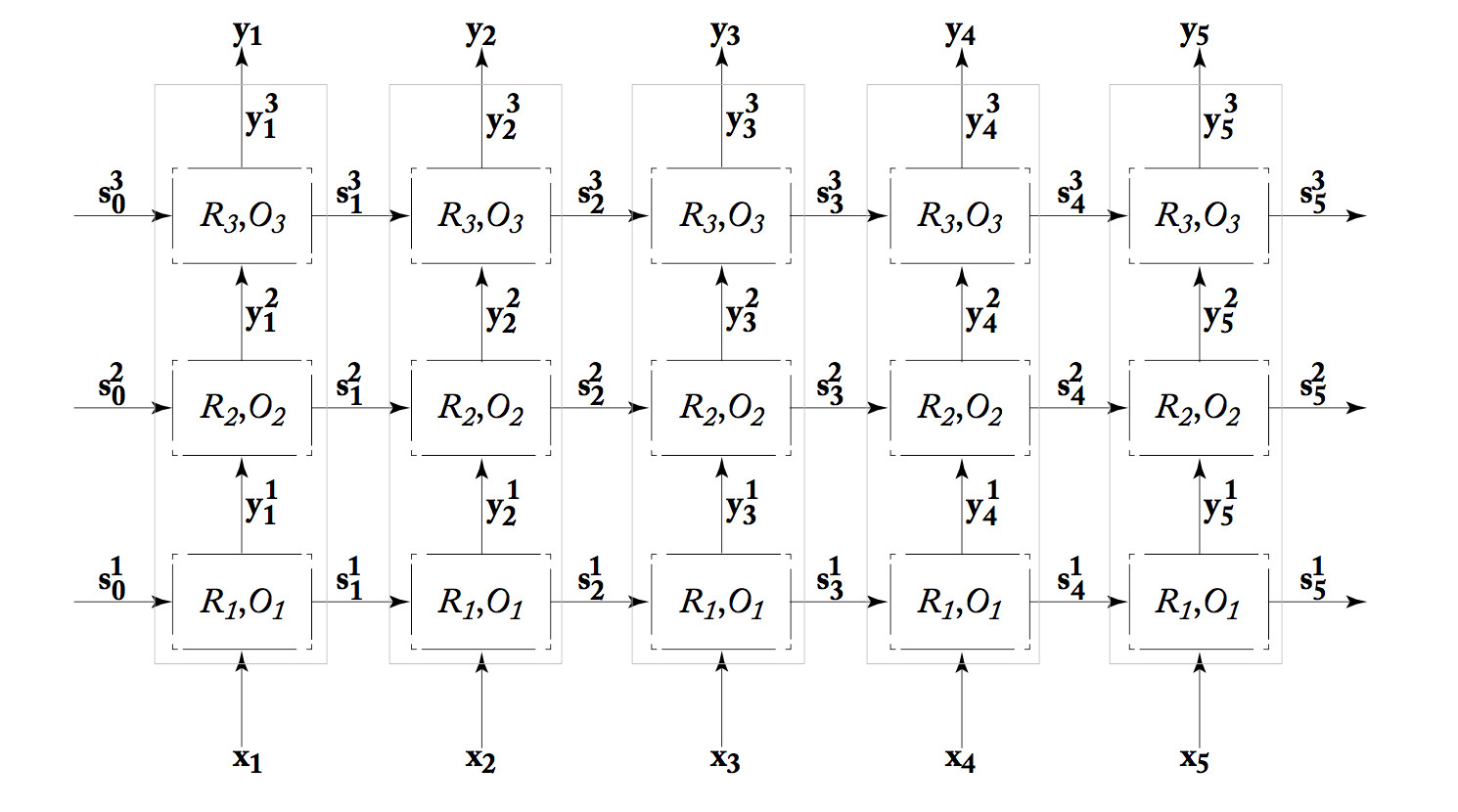from Yoav Goldberg

## Gated RNNs

In theory, an RNN can remember the entire stream of input values. However, gradient magnitude tends to decay as you move backwards from the loss signal. So earlier inputs may not contributed much to the RNN's final answer at the end. For a transducer, inputs may contribute little to the output for locations some distance away. This is a problem, because many linguistic tasks benefit from an extended context.

To allow RNNs to store information more effectively, researchers use "gated" versions of RNNs. These RNNs include separate vectors (the "gates") which control which parts of the unit's state will be updated at each timestep. The gates make the RNN's behavior easier to control, but create yet more tunable parameters that must be learned. Two popular gated RNN models are the "Long Short-Term Memory" (LSTM) and the "Gated Recurrent Unit" (GRU).

## Notes

Figures credited to Yoav Goldberg are from his book "Neural Network Methods for Natural Language processing." (If you're on the U. Illinois VPN, you can download it for free.)